Over a million developers have joined DZone.{{announcement.body}}
{{announcement.title}}

# Exploring the Gradient Descent Algorithm

DZone 's Guide to

# Exploring the Gradient Descent Algorithm

### In regression, we have data connecting a dependent variable and the variables it depends on, which are the independent variables. We want to find the equation between them that best represents the data, the best-fit equation.

· Big Data Zone ·
Free Resource

Comment (0)

Save
{{ articles.views | formatCount}} Views

Gradient Descent Algorithm is a key tool in Data Science used to find the minimum value of a function. You would have come across gradient in Microsoft PowerPoint or Adobe Photoshop when you start your slide or image creation. But what we are talking here is all about mathematical stuff, and to be specific - concepts from calculus.

The usage of gradient descent in data science is in regression. I’ve covered linear and logistics regression in my previous blog posts. To do a quick recap — linear regression is numeric prediction. Logistics Regression is binary category log-odds classification.

In regression, we have data connecting a dependent variable and the variables it depends on, which are the independent variables. We want to find the equation between them that best represents the data, the best-fit equation.

We start with an initial equation. Then improvise it. How do we do it? In the given data, for the known independent variable values, we calculate the value of the output variable as given by our initial equation. Then we compare these calculated values with the actual value of the output variable in the given data. The difference between the calculated value and the actual value is the error. Now the error values themselves collectively take the form of a function. Mathematicians / data scientists / nerds fondly call this as cost function. So our problem of finding the best-fit equation is one for which the error equation a.k.a cost function is minimum.

In order to find this minimum, we use calculus, in which the narrative starts with the derivative. Calculus is one of those subjects that will readily put you off. But then we need not comprehend the entire breadth of calculus, what we need to know is the derivative and the partial derivative. That calls for a detour into high school math.Let’s say x and y are the two variables. y is dependent on x. That is, the value of y is derived from the value of x by applying some formula on x. In other words, x is the input and y is the output or x is the independent variable and y is the dependent variable or y is a function of x. Mathematically, y = f(x).

The derivative is the rate of change of the function (y) at a specific value of x. When you say the rate at which something changes, it means it is a fraction or some part of the value is the change itself. To find the derivative we take a very small increment of the value of x, so small an increment that it approaches zero, and find the rate of change.

There is an opinion that the sensitivity is a better term than derivative. As one user expressed on Stack Overflow: ‘I dislike the word "derivative", which provides no hint of what a derivative is. My suggested replacement name is "sensitivity". The derivative measures the sensitivity of a function. In particular, it measures how sensitive the output is to small changes in the input. It is given by the ratio, where the denominator is the change in the input and the numerator is the induced change in the output. With this definition, it is not hard to show students why knowing the derivative can be very useful in many different contexts.’

In the physical world, the two best examples of derivatives are tangent and velocity. “The derivative of the position of a moving object with respect to time is the object's velocity: this measures how quickly the position of the object changes when time is advanced. The derivative of a function of a single variable at a chosen input value is the slope of the tangent line to the graph of the function at that point,” explains Wikipedia.

Now the thing is, will y be dependent only on one other variable, x? No. It could be dependent on or derived from multiple variables. So what do we do with the derivative then? No worries, humanity has this habit that when there are multiple variables, they focus only on one thing at a time. Here we take the derivative of y with respect to one variable (x) and keeping the other variables as constant. That is the partial derivative.

From partial derivatives, we go to the vector. Math.com explains it as “a vector is a specific mathematical structure. It has numerous physical and geometric applications, which result mainly from its ability to represent magnitude and direction simultaneously. Wind, for example, had both a speed and a direction and, hence, is conveniently expressed as a vector. The same can be said of moving objects and forces. The location of a point on a Cartesian coordinate plane is usually expressed as an ordered pair (x, y), which is a specific example of a vector. Being a vector, (x, y) has a certain distance (magnitude) from and angle (direction) relative to the origin (0, 0). Vectors are quite useful in simplifying problems from three-dimensional geometry.”

Now we come to the gradient. It is the short form of gradient vector. It is represented by the inverted triangle nabla. But nobody reads it as nabla. We just call it gradient.

The gradient of a function is the vector of partial derivatives of the function. For a function f(x, y), at a point P0 (x0, y0), it is obtained by evaluating the partial derivatives of f at P0 and summing them up.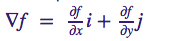For three variables it is,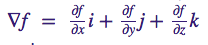and so on.

But what does it indicate? It points in the direction of steepest ascent and its magnitude is the slope of the steepest ascent. Good. We have a function and we know that its gradient gives the direction of the greatest increase, and its negative gives the direction of the greatest decrease. Time to get back to the cost function.

We take a small step down on our cost curve, check to see which direction we need to go to get closer to the minimum by using the negative of the gradient, and then take the next step to move closer to the minimum. We repeat until we get to the lowest point.

And that, my friends, is the gradient descent algorithm.

Let’s see all the mathematical symbols in their gradient glory

## The Derivative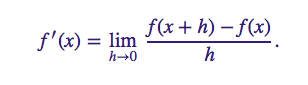ORVarious symbols used for the derivativeCost function in linear regressionCost function in logistic regression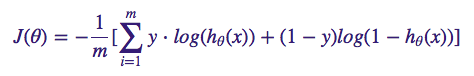Repeat until convergence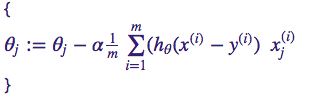Topics:
data science ,math

Comment (0)

Save
{{ articles.views | formatCount}} Views

Published at DZone with permission of

Opinions expressed by DZone contributors are their own.

# {{ parent.title || parent.header.title}}

{{ parent.tldr }}

{{ parent.urlSource.name }}